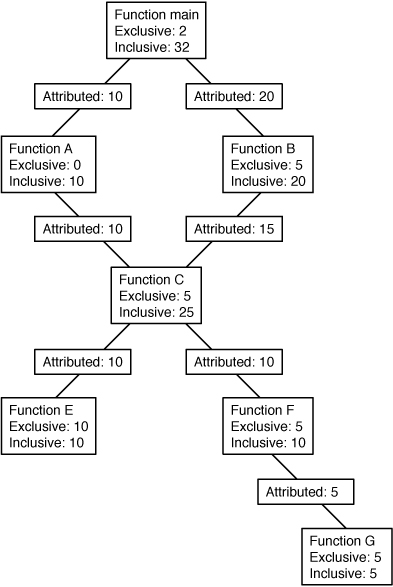Oracle® Solaris Studio 12.4: Performance Analyzer
Updated: January 2015

### Interpreting Attributed Metrics: An Example

Exclusive, inclusive and attributed metrics are illustrated in Figure 2–1, which contains a complete call tree. The focus is on the central function, function C.

Pseudo-code of the program is shown after the diagram.

Figure 2-1  Call Tree Illustrating Exclusive, Inclusive, and Attributed MetricsThe Main function calls Function A and Function B, and attributes 10 units of its inclusive metric to Function A and 20 units to function B. These are the callee attributed metrics for function Main. Their sum (10+20) added to the exclusive metric of function Main equals the inclusive metric of function main (32).

Function A spends all of its time in the call to function C, so it has 0 units of exclusive metrics.

Function C is called by two functions: function A and function B, and attributes 10 units of its inclusive metric to function A and 15 units to function B. These are the caller attributed metrics. Their sum (10+15) equals the inclusive metric of function C (25)

The caller attributed metric is equal to the difference between the inclusive and exclusive metrics for function A and B, which means they each call only function C. (In fact, the functions might call other functions but the time is so small that it does not appear in the experiment.)

Function C calls two functions, function E and function F, and attributes 10 units of its inclusive metric to function E and 10 units to function F. These are the callee attributed metrics. Their sum (10+10) added to the exclusive metric of function C (5) equals the inclusive metric of function C (25).

The callee attributed metric and the callee inclusive metric are the same for function E and for function F. This means that both function E and function F are only called by function C. The exclusive metric and the inclusive metric are the same for function E but different for function F. This is because function F calls another function, Function G, but function E does not.

Pseudo-code for this program is shown below.

```    main() {
A();
/Do 2 units of work;/
B();
}

A() {
C(10);
}

B() {
C(7.5);
/Do 5 units of work;/
C(7.5);
}

C(arg) {
/Do a total of "arg" units of work, with 20% done in C itself,
40% done by calling E, and 40% done by calling F./
}```